# Proof all one-, two- and three digit numbers reduce to zero

There are no single digit Eriam numbers because such numbers are their own reverse and therefore palindromes. The difference between a two-digit number and its reverse is simply the difference between the two digits of the number multiplied by(see this article to find out why). For example, the difference between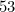and its reverse is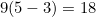So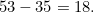Consider any two-digit number for which the difference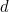between digits is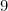(the number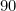is the only such number, but you’ll see what we’re getting at). Then the difference between that number and its reverse is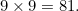The difference between digits for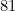is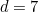, so the difference betweenand its reverse is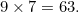Continuing in this vein gives

1.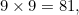2.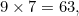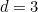3.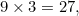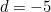4.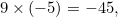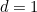5.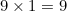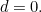This shows that a two-digit number with a difference of nine between the two digits will reduce to zero. Now suppose the difference between the digits is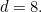For such numbers, the difference between number and reverse is

1.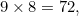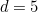This is effectively the third iteration from above, when the initialwas. The subsequent iterations will be the same, consequently. There is a change of sign due to a reversal of the digits, but that makes no difference to the outcome. So, any two-digit number with a digit-difference of eight will also reduce to zero.

This procedure can be carried out for the remaining possible values offrom one to seven. The first iteration for each of them will be one of the iterations for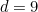above and consequently they will all reduce to zero.

The difference for three-digit numbers can be found in exactly the same way: take the difference between the first and last digits, but multiply it by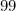, e.g. for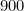,and

1.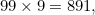2.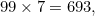3.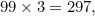4.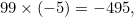5.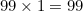The procedure will go through the same steps, with the same outcome, as for two-digit numbers, showing that all three-digit numbers reduce to zero.

Note thatis always odd. This is because the product of an integer (less than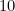) andhas first and last digits that sum to nine. This means that one digit is even and the other is odd. The difference between an odd and an even number is odd, sois odd.

Using a similar exhaustive technique it's quite easy to show that the first and the last 11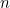-digit numbers, whenis greater than 3, always converge to zero. This also shows that 1012 must be the smallest Eriam number. Why not have a go at trying to prove it? You can send us your working by posting a comment to the main article.